#Number Systems Worksheets

## Dynamically Created Number Systems Worksheets

These Number Systems Worksheets are great for teaching working with different number systems. These different number based system are used in computer programming, engineering, and the information technologies industry. We have decimal to binary, octal, and hexadecimal worksheets as well as adding and subtracting binary and hexadecimal, and bitwise operations.

Click here for a Detailed Description of all the Number Systems Worksheets.

## Quick Link for All Number Systems Worksheets

Click the image to be taken to that Number Systems Worksheet.

#### Decimal and BinaryNumber Systems Worksheets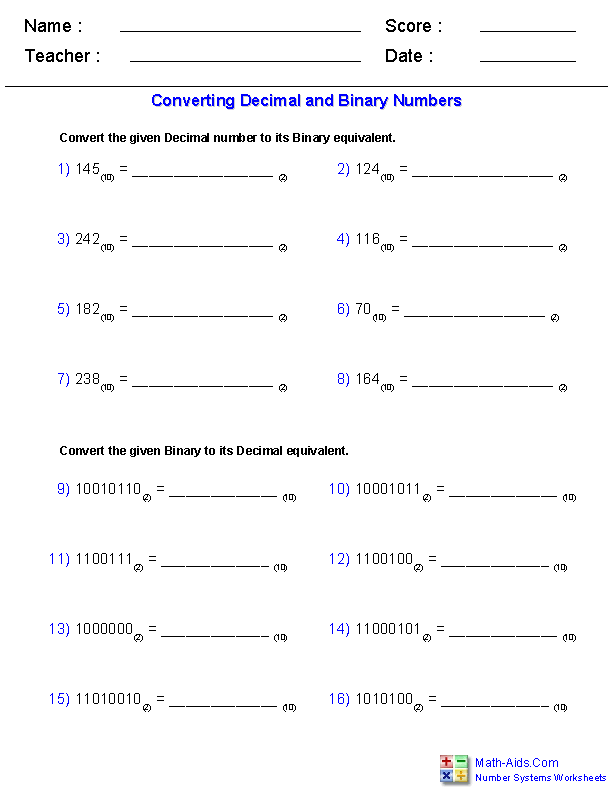#### Decimal and OctalNumber Systems Worksheets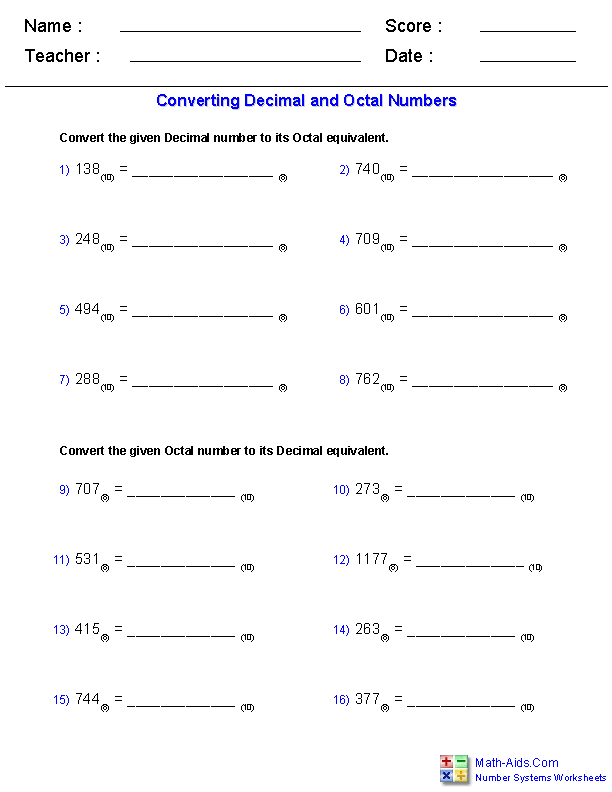#### Decimal and HexadecimalNumber Systems Worksheets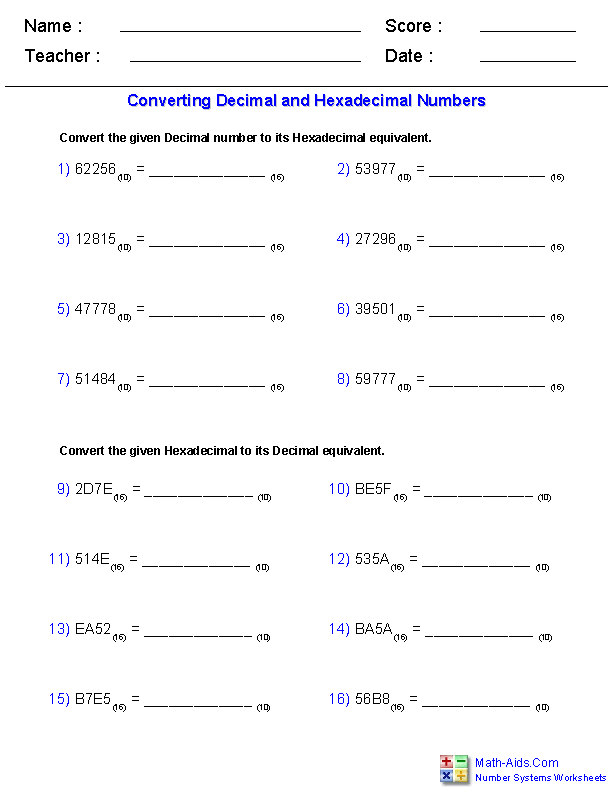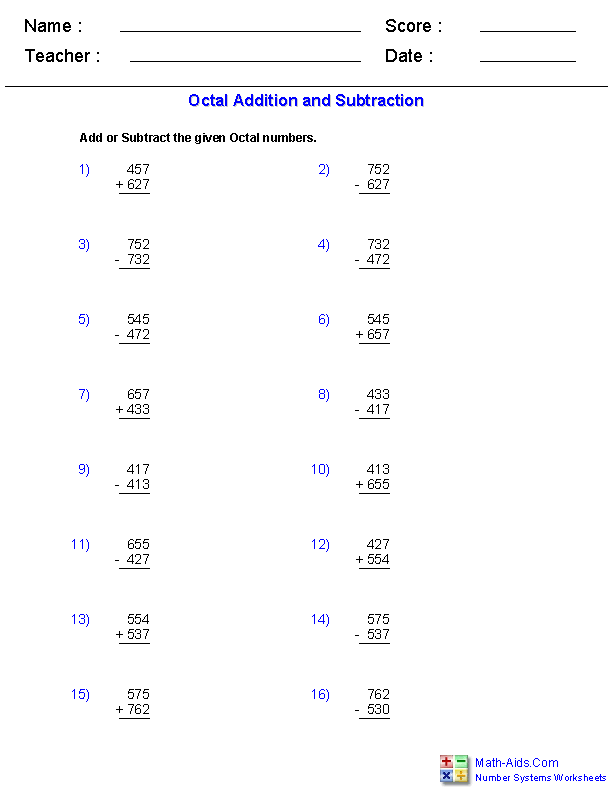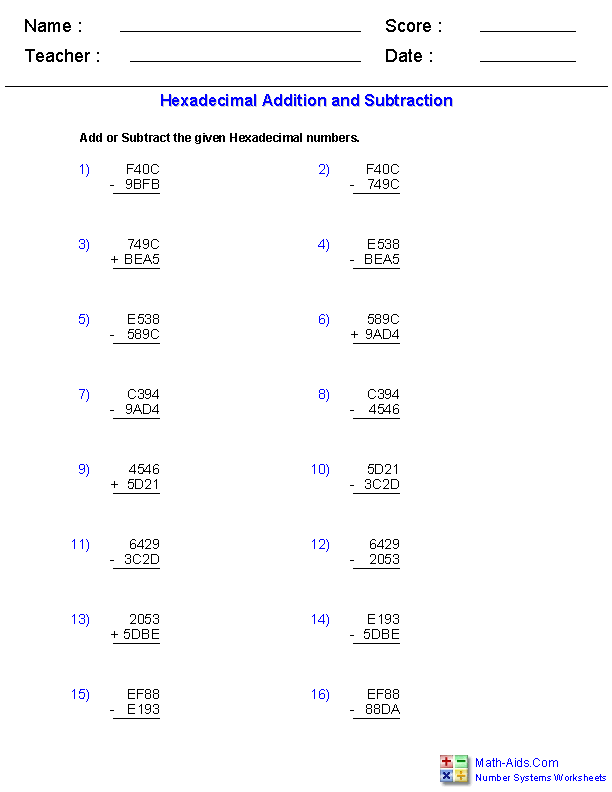#### Bitwise OperationsNumber Systems Worksheets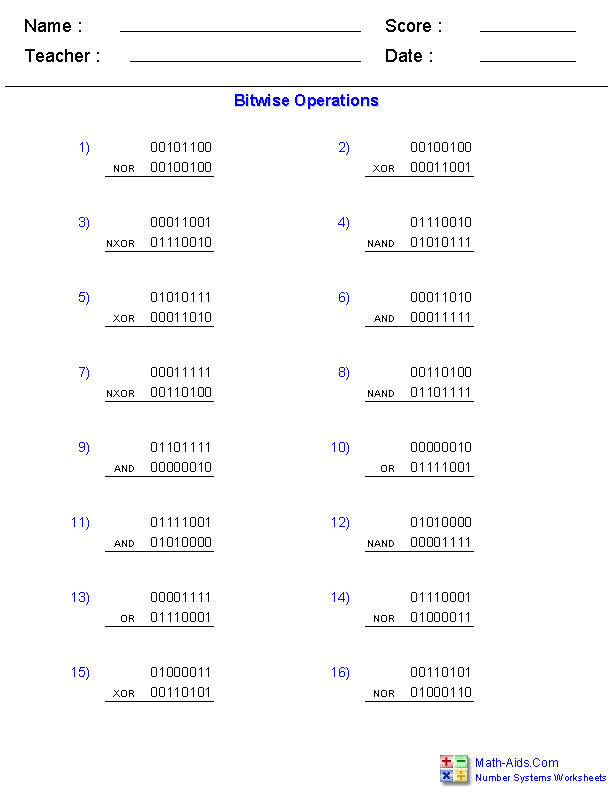#### Roman NumeralsNumber Systems Worksheets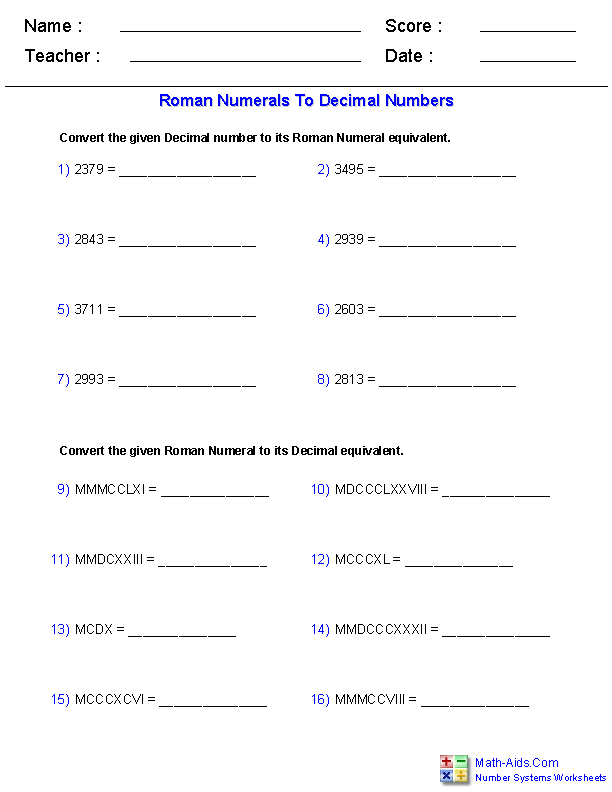Recommended Videos

## Detailed Description for All Number Systems Worksheets

Decimal and Binary Worksheets
These Number Systems Worksheets are great for working on converting numbers between decimal (Base 10) and binary (Base 2) number systems.

Decimal and Octal Worksheets
These Number Systems Worksheets are great for working on converting numbers between decimal (Base 10) and octal (Base 8) number systems.

These Number Systems Worksheets are great for working on converting numbers between decimal (Base 10) and hexadecimal (Base 16) number systems.

These Number Systems Worksheets are great for working on adding and subtracting binary (Base 2) numbers.

These Number Systems Worksheets are great for working on adding and subtracting octal (Base 8) numbers.

These Number Systems Worksheets are great for working on adding and subtracting hexadecimal (Base 16) numbers.

Bit Wise Operations Worksheets
These Number Systems Worksheets are great for working with the basic logic operators, AND, OR, & XOR. These bit wise operators are used in engineering and computer programming.

Roman Numerals Worksheets
These Number Systems Worksheets are great for working on converting numbers between decimal (Base 10) and Roman Numerals.

If you are trying to learn Roman numerals, or just need quick results with this ancient number system, be sure to check out these Roman numerals resources. Each Roman numeral chart is formatted to print precisely on a page and this visual Roman numeral converter provides an interactive view of how Roman numerals are constructed.